### Home > INT2 > Chapter 12 > Lesson 12.2.2 > Problem12-78

12-78.
1. Each of the petit fours (tiny bite-sized layered cakes) at Pauline’s Pastries are made with three different layers of filling. The pastry chef has eight different choices for fillings. These cakes are eaten in one bite, so the order of the fillings does not matter. Homework Help ✎

1.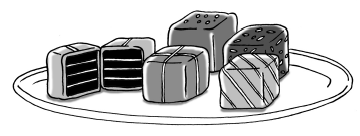If the pastry chef makes one of each of the possible petit fours, how many petit fours can the chef make?

2. How many have raspberry, custard, and one other filling?

3. What is the probability (as a percent) of getting a petit four that has apricot filling?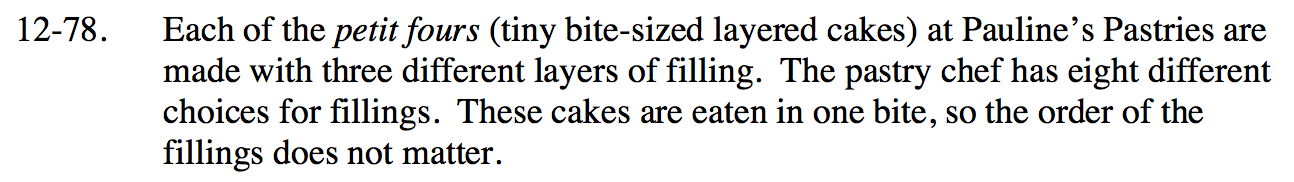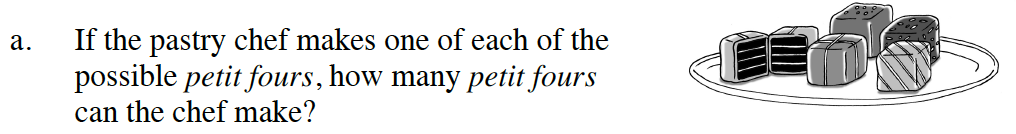Refer to the Math Notes box.

8C3 = 56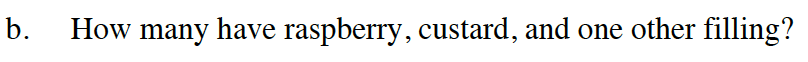How many other filling choices are left?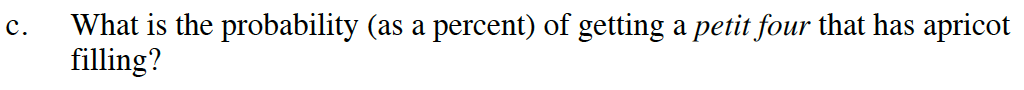$\frac{\# \text{of apricot-filled petit fours}}{\text{total \# } \text{of petit fours}}$

$\frac{_7C_2}{56}$